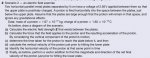# Physics: Electric Fields and Projectile Motion

#### miaellenaylee

##### New member
Hi,
I required some help with the question below. I was quite stumped when i reached this part of the question. I'm not sure sure where to start.

QUESTION:
Two horizontal parallel metal plates are separated by 5cm have a voltage of 2.56V applied between them so that the upper plate is positively charged. A proton is fired horizontally into the space between the plates, just below the upper plate. Assume the plates are large enough that the proton will remain in . the space and ignore any gravitational effects.

Data: mass of proton = 1.67 x 10^-27 kg
Charge of proton = 1.60 x 10^-19 C

e) Identify the horizontal velocity of the proton at prior to hitting the lower plate.

Data I have found from the other sections of the question:
Electric Field Strength: 51.2 NC^-1
acceleration: 4.91 x 10^9 m/s^2 down
time to reach lower plate: 4.52 x 10^-6 sec or 4.52µs
Vertical velocity: 2.21 x 10^4 m/s

All I know is a=0 in the horizontal.

Last edited by a moderator:

#### Subhotosh Khan

##### Super Moderator
Staff member
Hi,
I required some help with the question below. I was quite stumped when i reached this part of the question. I'm not sure sure where to start.

QUESTION:
Two horizontal parallel metal plates are separated by 5cm have a voltage of 2.56V applied between them so that the upper plate is positively charged. A proton is fired horizontally into the space between the plates, just below the upper plate. Assume the plates are large enough that the proton will remain in . the space and ignore any gravitational effects.

Data: mass of proton = 1.67 x 10^-27 kg
Charge of proton = 1.60 x 10^-19 C

e) Identify the horizontal velocity of the proton at prior to hitting the lower plate.

Data i have found from the other sections of the question:
Electric Field Strength: 51.2 NC^-1
acceleration: 4.91 x 10^9 m/s^2 down
time to reach lower plate: 4.52 x 10^-6 sec or 4.52µs
Vertical velocity: 2.21 x 10^4 m/s

All I know is a=0 in the horizontal.
This one I am going leave for Dan (topsquark).......

Staff member

#### topsquark

##### Full Member
Hi,
I required some help with the question below. I was quite stumped when i reached this part of the question. I'm not sure sure where to start.

QUESTION:
Two horizontal parallel metal plates are separated by 5cm have a voltage of 2.56V applied between them so that the upper plate is positively charged. A proton is fired horizontally into the space between the plates, just below the upper plate. Assume the plates are large enough that the proton will remain in . the space and ignore any gravitational effects.

Data: mass of proton = 1.67 x 10^-27 kg
Charge of proton = 1.60 x 10^-19 C

e) Identify the horizontal velocity of the proton at prior to hitting the lower plate.

Data I have found from the other sections of the question:
Electric Field Strength: 51.2 NC^-1
acceleration: 4.91 x 10^9 m/s^2 down
time to reach lower plate: 4.52 x 10^-6 sec or 4.52µs
Vertical velocity: 2.21 x 10^4 m/s

All I know is a=0 in the horizontal.
The electric field in between the capacitor plates only has a vertical component. Thus the proton is not accelerated in the horizontal direction thus the horizontal component of the velocity of the proton is constant.

So to find the horizontal component of the velocity we need only know what it was when the proton was first projected between the plates. We don't have the information listed to figure that out. Is this the whole problem statement?

Also, what do you mean by "vertical velocity?" The proton accelerates toward the negative plate and thus the velocity changes. Is this, perhaps, the speed when the proton reaches the negative plate?

Please give us the whole question.

-Dan

#### miaellenaylee

##### New member
The electric field in between the capacitor plates only has a vertical component. Thus the proton is not accelerated in the horizontal direction thus the horizontal component of the velocity of the proton is constant.

So to find the horizontal component of the velocity we need only know what it was when the proton was first projected between the plates. We don't have the information listed to figure that out. Is this the whole problem statement?

Also, what do you mean by "vertical velocity?" The proton accelerates toward the negative plate and thus the velocity changes. Is this, perhaps, the speed when the proton reaches the negative plate?

Please give us the whole question.

-DanFor this particular question, I think the vertical velocity is the at speed the proton is travelling at prior to hitting or reaching the plate below so yes your interpretation is correct. I have attached the entire question as an image, I hope you can viewed it. Part E is the section I required help with.#### Subhotosh Khan

##### Super Moderator
Staff member
View attachment 10875

For this particular question, I think the vertical velocity is the at speed the proton is travelling at prior to hitting or reaching the plate below so yes your interpretation is correct. I have attached the entire question as an image, I hope you can viewed it. Part E is the section I required help with.Have you done any work with the parts (a), (b), etc.? If you did - please share your work - so that we know where to begin to help you!!